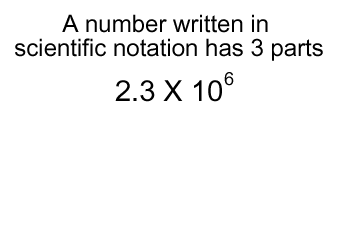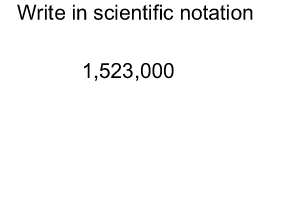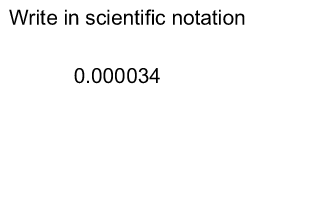Scientific notation

Scientific notation is based on powers of ten.

The number 2,300,000 is written as 2.3 X 106 .
Note. The first number 2.3 is called the coefficient and it must be between 1 and 10. Look at the animation on the right.The speed of light is 300,000km/s. We can write this number as 3.0 X 105km/s

The mass of a carbon atom is 0.000000000000000000000002 grams. We can write this as 2.0 X 10-23 grams.

The number of hydrogen atoms in 1 gram of hydrogen is 623,000,000,000,000,000,000,000. We can write this as 6.23 X 1023 .

You can see why we write in scientific notation.

To write a number in scientific notation we take two steps.

Step 1 Move the decimal point to the left or right so that the coefficient is between 1 and 10.

Step 2 For every decimal place the decimal point moves left or right we add or subtract 1 from the exponent. When the decimal point moves to the left we add 1 to the exponent. When the decimal point moves right we subtract 1 from the exponent. Look at the animation on the right.Express 0.000034 in scientific notation. Look at the animation on the right.Express 1,234,000 in scientific notation. Solution
Express 123.45 X 106 in scientific notation. Solution
 Number Scientific notation? 124.78 Yes No 2.3 X 104 Yes No 0.3 X 1010 Yes No 12.3 X 1014 Yes No 1.4 X 104 Yes No 9.9 X 1012 Yes No Solution Continue